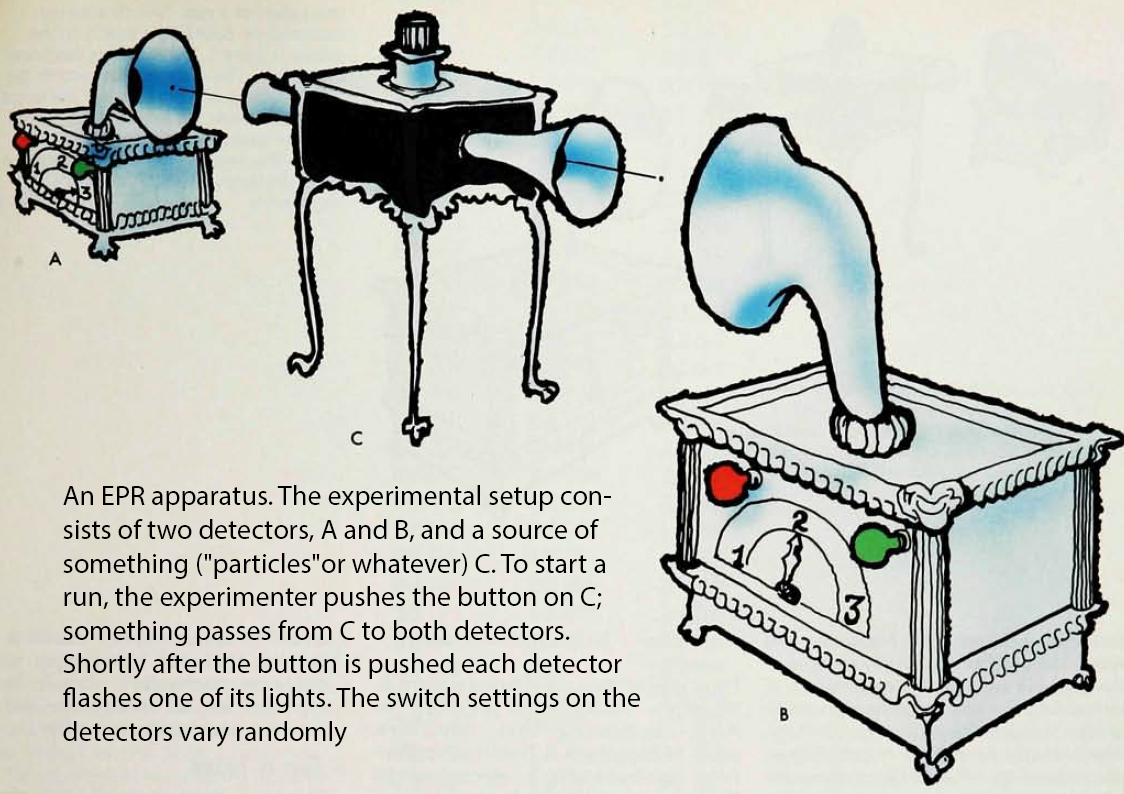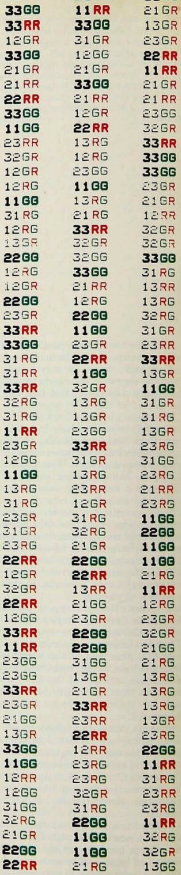Citation for this page in APA citation style.

N. David Mermin
David Mermin is professor emeritus of physics at Cornell University. He is perhaps best known for his contributions to the foundations of physics, especially his mechanisms for describing Bell's Theorem, his contributions to quantum information science, and his defense of QBism.

In !985, Mermin wrote the very popular and widely cited paper "Is the Moon There When Nobody Looks?" in Physics Today.

In the paper Mermin described what he called a "very simple version" of John Bell's gedanken experiment. Mermin says his "EPR Apparatus" exhibits all the experimental behavior of Bell's version, without any reference to the "underlying mechanism that makes the gadget work." He provides samples of (hypothetical) data produced by the apparatus, which presumably matches (statistically) the data produced in real experimental tests of Bell's Theorem.A few years later, Mermin published a variation on his original apparatus at a 1987 Notre Dame conference on Bell's Theorem. In this work, "More Experimental Physics from EPR," his new device has different switch settings but more data is provided to exhibit the mysterious entanglement (perfect correlations) between widely separated measurements. In this paper, Mermin gave a definite answer to his earlier question about the moon, "We now know that the moon is demonstrably not there when nobody looks." (p.50)

Perfect Correlations Depend on Polarizer Angles
 In real EPR tests (e.g., the Aspect experiment), the settings of polarizers at A and B are adjusted to a few specific angles, for example 0°, 22.5°, 45°, 90°, 135°. The angles chosen are expected to show the greatest differences between Bell's inequality predictions and quantum mechanics. In particular, when the polarizers measure the same angle, so their angle difference is 0°, the photon spin correlation (or anti-correlation for spin-1/2 electrons) is perfect. In Mermin's EPR device, different polarizer angles are represented by different switch settings on the detectors (11, 12, 13, 21, 22, 23, 31, 32, 33). But he does not tell us the angle difference between the measurements. Mermin's cases of "switches set the same" correspond to detectors measuring the same angle. For Mermin's device, lights flashing the same color (RR or GG) shows the correlations are perfect. When polarizers are set at different angles, it is well known that the transmission of light falls off as the square of the cosine of the angle difference θ, cos2θ. This is known as the "law of Malus." At 45 degrees, only half of the photons pass through. The other half is absorbed. At 0° all pass through. At 90° all are absorbed. For Merlin's device, when lights are different colors, there is a loss of correlation. Mermin's hypothetical data illustrate the fact that at some polarizer angles (when detector switches are set the same) the correlation between measurements is perfect. (For Bohm's electron spins it is perfect anti-correlation - if one spin is up, the other is down.) Mermin's data are for Aspect spin-1 photons, which are initially entangled with spins in the same direction.) Mermin divides his results into two cases: Case (a). In those runs in which each switch ends up with the same setting (11,22, or 33) both detectors always flash the same color: RR and GG occur with equal frequency; RG and GR never occur. Case (b). In those runs in which the switches end up with different settings (12,13, 21, 23, 31, or 32) both detectors flash the same color only a quarter of the time (RR and GG occurring with equal frequency); the other three quarters of the time the detectors flash different colors (RG and GR occurring with equal frequency). (Mermin, 1981, p.941) The quantum physics behind the reduced correlations in Mermin's case (b) is that light passing through polarizers falls off as the square of the cosine of the angle between the polarizers. This is the "law of Malus." See Stuckey et al (2020). In his classic book Principles of Quantum Mechanics, Paul Dirac describes photons in terms of his quantum state vectors. He tells us a diagonally polarized photon can be represented as a superposition of vertical | v > and horizontal | h > quantum states, with complex number coefficients 1/√2 that represent "probability amplitudes," which are squared to get probabilities. Thus, a diagonally polarized photon is | d > = ( 1/√2) | v > + (1/√2) | h >          (1) Dirac illustrates thisThe physical meaning of a superposition of quantum states is that in a large number of identical experiments, the probability of a | d > photon passing through a vertical polarizer is 1/2. Switches set the same: highlighted to pick out those runs in which both detectors had the same switch settings as they flashed. Note that in such runs the lights always flash the same colors.Can Perfect Correlations Be Explained by Conservation Laws?
David Bohm, Eugene Wigner, and even John Bell suggested that conservation of angular momentum (or particle spin) tells us that if one spin-1/2 electron is measured up, the other must be down. Albert Einstein used conservation of linear momentum in his development of the EPR Paradox.

David Bohm wrote in 1957,

We consider a molecule of total spin zero consisting of two atoms, each of spin one-half. The wave function of the system is therefore

ψ = (1/√2) [ ψ+ (1) ψ- (2) - ψ- (1) ψ+ (2) ]

where ψ+ (1) refers to the wave function of the atomic state in which one particle (A) has spin +ℏ/2, etc. The two atoms are then separated by a method that does not influence the total spin. After they have separated enough so that they cease to interact, any desired component of the spin of the first particle (A) is measured. Then, because the total spin is still zero, it can immediately be concluded that the same component of the spin of the other particle (B) is opposite to that of A.

Eugene Wigner wrote in 1962

If a measurement of the momentum of one of the particles is carried out — the possibility of this is never questioned — and gives the result p, the state vector of the other particle suddenly becomes a (slightly damped) plane wave with the momentum -p. This statement is synonymous with the statement that a measurement of the momentum of the second particle would give the result -p, as follows from the conservation law for linear momentum. The same conclusion can be arrived at also by a formal calculation of the possible results of a joint measurement of the momenta of the two particles.

Writing a few years after Bohm, and one year before Bell, Wigner explicitly describes Einstein's conservation of momentum example as well as the conservation of angular momentum (spin) that explains perfect correlations between angular momentum (spin) components measured in the same direction
One can go even further: instead of measuring the linear momentum of one particle, one can measure its angular momentum about a fixed axis. If this measurement yields the value mℏ, the state vector of the other particle suddenly becomes a cylindrical wave for which the same component of the angular momentum is -mℏ. This statement is again synonymous with the statement that a measurement of the said component of the angular momentum of the second particle certainly would give the value -mℏ. This can be inferred again from the conservation law of the angular momentum (which is zero for the two particles together) or by means of a formal analysis.

John Bell wrote in 1964,

With the example advocated by Bohm and Aharonov, the EPR argument is the following. Consider a pair of spin one-half particles formed somehow in the singlet spin state and moving freely in opposite directions. Measurements can be made, say by Stern-Gerlach magnets, on selected components of the spins σ1 and σ2. If measurement of the component σ1a, where a is some unit vector, yields the value + 1 then, according to quantum mechanics, measurement of σ2a must yield the value — 1 and vice versa. Now we make the hypothesis, and it seems one at least worth considering, that if the two measurements are made at places remote from one another the orientation of one magnet does not influence the result obtained with the other.
"pre-determination" is too strong a term. The first measurement just "determines" the later measurement. We shall see that the second measurement is synchronous with the "first" in a "special" frame
Since we can predict in advance the result of measuring any chosen component of σ2, by previously measuring the same component of σ1, it follows that the result of any such measurement must actually be predetermined.

Since the initial quantum mechanical wave function does not determine the result of an individual measurement, this predetermination implies the possibility of a more complete specification of the state.

Just like Bohm and Wigner, Bell is implicitly using the conservation of total spin.

Albert Einstein made the same argument in 1933, shortly before EPR, though with conservation of linear momentum, asking Leon Rosenfeld,

Suppose two particles are set in motion towards each other with the same, very large, momentum, and they interact with each other for a very short time when they pass at known positions. Consider now an observer who gets hold of one of the particles, far away from the region of interaction, and measures its momentum: then, from the conditions of the experiment, he will obviously be able to deduce the momentum of the other particle. If, however, he chooses to measure the position of the first particle, he will be able tell where the other particle is.

Supporters of the Copenhagen Interpretation (including Mermin?) claim that the properties of the particles (like angular or linear momentum) do not exist until they are measured. It was Pascual Jordan who claimed the measurement creates the value of a property. This is true when the preparation of the state is in an unknown linear combination (superposition) of quantum states.

In our case, the entangled particles have been prepared in a superposition of states, both of which have total spin zero.

ψ = (1/√2) [ ψ+ (1) ψ- (2) - ψ- (1) ψ+ (2) ]

So whichever of these two states is created by the preparation, it will put the two particles in opposite spin states, randomly + - or - + , but still supporting the views of Bohm, Wigner, and Bell, that they will be perfectly (anti-)correlated when measured.

Wolfgang Pauli called it a "measurement of the first kind" when a system is prepared in a state and if measured again, will be certainly found in the same state. (This is the basis for the quantum zeno effect.)

As long as nothing interferes with either entangled particle as they travel to the distant detectors, they will be found to be perfectly correlated if (and only if) they are measured at the same angle. Otherwise. the correlations should fall off as the square of the cosine of the angle difference. Oddly, Bell's inequality predicts a linear falloff with the angle difference, and a strange non-physical "kink" at angles 0°, 90°, 180°, and 270° (which Bell himself pointed out).

References
"Bringing home the atomic world:Quantum mysteries for anybody." American Journal of Physics 49(10) (1981): 940-943.

"Is the Moon There When Nobody Looks? Reality and the Quantum Theory." Physics Today 38.4 (1985): 38-47.

"More Experimental Physics from EPR," in Philosophical Consequences of the Quantum Theory, Reflections on Bell's Theorem, J.T.Cushing and E. McMullin, eds. Notre Dame, 1989. pp. 38-59

"What is Quantum Mechanics Trying to Tell Us?," American Journal of Physics 66.9 (1998): 753-767.

"An Introduction to QBism," American Journal of Physics 82.8 (2014): 749-754.

"Answering Mermin’s Challenge with Conservation per No Preferred Reference Frame," Stuckey, W, Silberstein, M, McDevitt, T. and Le, T.D. (2020) researchgate.net

Normal | Teacher | Scholar